# -1.序言

• 想了解更多关于数论的内容，可戳这里(灵感所在)，这是出处

# 0、由来

$\begin{cases}a_{11}x_1+a_{12}x_2+...+a_{1n}x_n=b_1\\a_{21}x_1+a_{22}x_2+...+a_{2n}x_n=b_2\\. . .\\. . .\\. . .\\a_{n1}x_1+a_{n2}x_2+...+a_{nn}x_n=b_n\end{cases}$

• 未知量较多

• 方程个数不同

• 是否有解？

• 如果有解，是否唯一？

• 如果不唯一，解有什么规律与结构？

# 1、高斯消元

#### 高斯：

$\begin{cases}a_{11}x_1+a_{12}x_2+...+a_{1n}x_n=b_1\\a_{21}x_1+a_{22}x_2+...+a_{2n}x_n=b_2\\. . .\\. . .\\. . .\\a_{n1}x_1+a_{n2}x_2+...+a_{nn}x_n=b_n\end{cases}$

$\begin{cases}x_1+3x_2+4x_3=5\\x_1+4x_2+7x_3=3\\9x_1+3x_2+2x_3=2\end{cases}$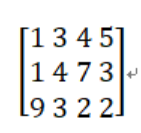## 第一步：对左侧的系数进行消元，即对：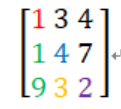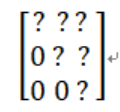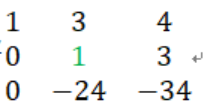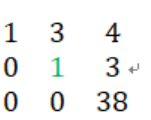Q：这里不会把之前第一列化好的0给冲掉吗？

A:显然，在上一步已经保证第一列除主元外系数皆为0，所以不会出现上述情况

Q:万一上面那个绿1为0怎么办？

A:这就是比较特殊的主元为0的情况，此时因为每一个方程地位都相等，所以只需将主元所在的行与下方主元位置非0的行互换即可。

A:那么方程就无解了，这种情况在后面也会提到。## 第二步：对增广矩阵消元## 第三步：回带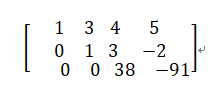$\begin{cases}x_1+3x_2+4x_3=5\\0x_1+1x_2+3x_3=-2\\0x_1+0x_2+38x_3=-91\end{cases}$

$\begin{cases}x_1+3x_2+4x_3=5\\1x_2+3x_3=-2\\38x_3=-91\end{cases}$

$$x_3=\frac{-91}{38}=-2.39$$ （保留了两位小数）

$$x_2=5.18$$

$$x_1=-0.97$$

### 于是，对高斯消元的手动模拟就结束了QAQ

#include<cstdio>
#include<cmath>
#include<iostream>
using namespace std;
const double eps=1e-7 ;
double a,ans;
int main()
{
int n;
scanf("%d",&n);
for(int i=1;i<=n;i++)
{
for(int j=1;j<=n+1;j++)//这里千万别忘了右侧那一列了
{
scanf("%lf",&a[i][j]);
}
}
for(int i=1;i<=n;i++)
{
int m=i;
for(int j=i+1;j<=n;j++)
if(fabs(a[m][i])<fabs(a[j][i]))//fabs能取浮点数绝对值
m=j;//找到当前这一列最大的数字,作为主元消元，这样能最大限度的避免精度误差
if(fabs(a[m][i])<eps)//这里要判精度，其实就是如果该位置的系数为0则无解（double没法准确处理这种精度
{
printf("No Solution\n");
return 0;
}
if(i!=m)swap(a[i],a[m]);//如果巧了，当前行不是最大行，那得罪了，你换下去吧，让未来的主元上来，好直接枚举之后的方程就行了
double d=a[i][i];
for(int j=i;j<=n+1;j++)
a[i][j]/=d;//将该位置的系数变为1
for(int j=i+1;j<=n;j++)
{
d=a[j][i];
for(int k=i;k<=n+1;k++)
{
a[j][k]-=a[i][k]*d;//将其他的方程用两式相减的方法减去应当减掉的系数的值
}
}
}
ans[n]=a[n][n+1];
for(int i=n-1;i>=1;i--)//回带，最后一行直接写出答案，然后其他行还有等待前面处理出来，从后往前推即可
{
ans[i]=a[i][n+1];//这里不难理解
for(int j=i+1;j<=n;j++)
{
ans[i]-=a[i][j]*ans[j];
}
}
for(int i=1;i<=n;i++)
{
printf("%.2lf\n",ans[i]);
}
return 0;
}

## 大概就是：

$$q_{jn}=q_{jn}-\frac{q_{j1}}{q_{i1}}\times q_{iw}$$

# 2、高斯——约旦消元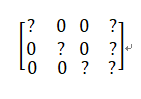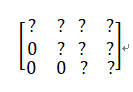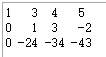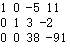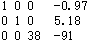$\begin{cases}x_1+0x_2+0x_3=-0.97\\0x_1+x_2+0x_3=5.18\\0x_1+0x_2+38x_3=-91\end{cases}$

$\begin{cases}x_1=-0.97\\x_2=5.18\\38x_3=-91\end{cases}$

$\begin{cases}x_1=-0.97\\x_2=5.18\\x_3=-2.39\end{cases}$

#include<cstdio>
#include<cmath>
#include<iostream>
using namespace std;
double a;
int main()
{
int n;
scanf("%d",&n);
for(int i=1;i<=n;i++)
{
for(int j=1;j<=n+1;j++)
{
scanf("%lf",&a[i][j]);
}
}
for(int i=1;i<=n;i++)//枚举列（项）
{
int m=i;
for(int j=i+1;j<=n;j++)//选出该列最大系数
{
if(fabs(a[j][i])>fabs(a[m][i]))//fabs是取浮点数的绝对值的函数
{
m=j;
}
}
for(int j=1;j<=n+1;j++)//交换
{
swap(a[i][j],a[m][j]);
}
if(a[i][i]==0)//最大值等于0则说明该列都为0，肯定无解
{
printf("No Solution\n");
return 0;
}
for(int j=1;j<=n;j++)//每一项都减去一个数
{
if(j!=i)//不是主元那一项
{
double d=a[j][i]/a[i][i];
for(int k=i+1;k<=n+1;k++)
{
a[j][k]-=a[i][k]*d;
}
}
}
}
for(int i=1;i<=n;i++)//最后的结果系数可能不为1，所以记得消去常数
{
printf("%.2lf\n",a[i][n+1]/a[i][i]);
}
return 0;
}

# 3、例题

## 例题1：

P2455 [SDOI2006]线性方程组

• 无实数解输出-1
• 无穷多实数解输出0
• 有唯一解，则输出解（小数点后保留两位小数）。具体格式见样例

# 分析：

（我们知道，只要一个未知数无解或多解就可以说明整个方程的情况）：

### 无解：$if(a[i][i]==0$&& $a[i][n+1]!=0)$无解

### 多解：$if(a[i][i]==0$&& $a[i][n+1]==0)$多解

• 要注意先判断无解，如果是的话直接跳出，然后轮到多解。

## 例题2:

（这题我没有找到出处）

### 题目：【维他命的配方】

#### 输入样例：

4
100 200 300 400
4
50 50 50 50
30 100 100 100
20 50 150 250
50 100 150 200

#### 输出样例：

YES
1 1 1 0

# 分析：

$\begin{cases}50x_1+30x_2+20x_3+50x_4=100\\50x_1+100x_2+50x_3+100x_4=200\\50x_1+100x_2+150x_3+150x_4=300\\50x_1+100x_2+250x_3+200x_4=400\end{cases}$

• 这第一步很好想到，但是行列的顺序却容易弄错，仔细观察就会发现需要把 $V_i$补到矩阵的第G+1行，然后每一列为一个方程计算。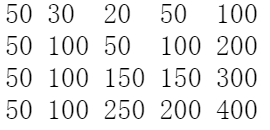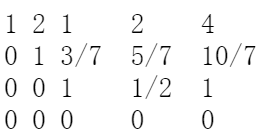（呀这怎么办！）（刚才的知识怎么学的！不就是个多解情况吗？！）

# 4、高斯消元注意事项：

• 模板不要背错
• 为了避免精度问题，最好和eps比较而不是和0比较（虽然有时这个基本相同）
• 无解多解的判断情况以及顺序
• 遇到题目转换成高斯消元时不要兴奋的转换出错或弄反